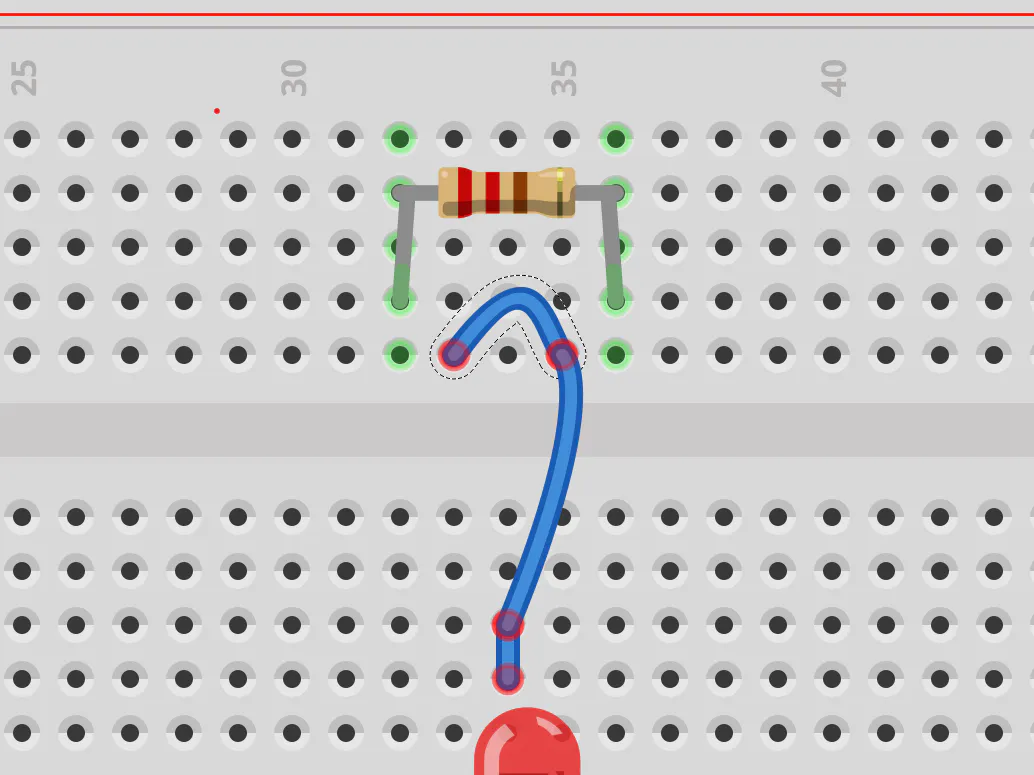# Finally a way to sort out your resistors © GPL3+

OK! There's the color codes but small as they are we can use a simple "ohmmeter" that just gives us the value with a single move.

• 3,351 views
• 2 comments
• 11 respects

## Components and suppliesArduino UNO
×1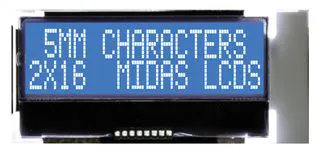Alphanumeric LCD, 16 x 2
×1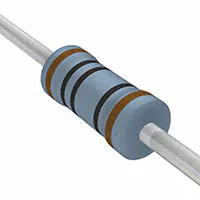Resistor 100 ohm
×1Resistor 1k ohm
×1Resistor 10k ohm
×1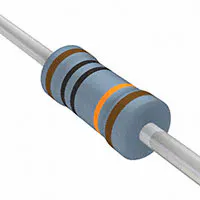Resistor 100k ohm
×1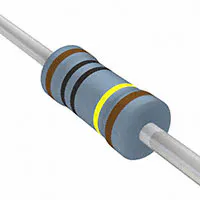Resistor 1M ohm
×1Jumper wires (generic)
×1

## About this project

The "demand" I address with this work is reading the value of a resistor in a simple way to sort them back in the proper drawers and keep the table uncluttered. Yes, there's the color codes but... ;-( YES, there's the multimeter with the proper attachments but...

Is it not better to just plainly READ IT?

I started this first project as a way to learn better and make it available a great idea to those who have just started with a basic kit.

So I started this first project as a way to learn better and make it available a great idea (Federico's - see credits) to those who have just started with a basic kit and the UNO board.

The theoretical principle that is applied here (and in many other projects) is the "Voltage divider equation" (see Wikipedia https://en.wikipedia.org/wiki/Voltage_divider) - to make difficult things simple it is a way to calculate one resistor value that you don't know putting two one after the other and reading how much of the total voltage the know resistor make you read at its terminals. The nearer the two are the more precise the result can be - so to make a balanced compromise we use five different values of know sample resistors.

Let's get started!!!

You need to make ready the components. The scheme below will easily take you to configure the breadboard. I used two different ones - to keep the 16x2 LED display function as much similar as to those of the tutorials (you should try the display as a separate part first, if you have not done it yet!) and get more visibility on the simple resistor part 'addition'. There's plenty of space left on the main breadboard to move it all on one - you just need to keep connected with the 5 green wires and the yellow one as before.

When you have arranged the components in this way you can also try to move it around checking with the schematics below:

At the end it should be something like...

Now is time to open up the coding - pick up the code in the proper section of this page and start reading it to feel it up. As everything in an Open Source environment it is a collage of what others have built before us. In particular all the sections that enable the visualization on the LCD is very standard.

I added on the original project a FUNCTION - that is a separate piece of code that gets AFTER the usual two sections of "setup" and "loop" - that can be "called" to make a specific calculation or - in the case a formatting - of one or more variables. I used it to transform a number 1.000.121, 34 in a string that is readable after the normal conventions for resistors - such as 1MOhm. The rounding and formatting would need a more extensive testing to check for all exceptions and anomalies... help me there.

String OhmFormattingFunction(float Input) {

//FS 20200223 v0 - Ohm values formatting function **

String Result;

if (Input < 80) {

valore = (String(round(Input)));

suffisso = " ";

}

....

I confess I kept some words inside the code in the Italian (Leonardo's!) language but it will take just a moment for you to replace them with... whatever idiom you were born to or in the English we all share. There are extensive debugging inserts in form of printed serial text to follow the procedure from the monitor.

IMPROVEMENT PATH:

• First of all you should make this yours by adapting it to your style and needs - correct the unavoidable mistakes and try to improve the calculations and/or the formatting letting us all know by commenting (much appreciated)
• I strongly suggest you buy a 0.96" OLED display and try it out - it is quite inexpensive and easy to implement (see Federico's project)
• Another way to get nearer to a finished "product" is to port it to a Nano Every - I found that the pins are the same etc. (this requires soldering and although it is not difficult, it implies a different level of involvement).

## Code

##### lazyRmeter_v3_16x2.inoArduino
```// lazyRmeter by FEDERICO VIVALDI all references to OLE display removed - pins relocated
// lazyRmeter_v2_16x2 semplified by F.Stella 20200221 for UNO and serial print to be ported to simple 16x2 display

// LCD 16x2 code - example added 9 Jul 2009 by Tom Igoe - modFS2020
//include the LCD library code:
#include <LiquidCrystal.h>

// initialize the library by associating any needed LCD interface pin
// with the arduino pin number it is connected to
const int rs = 12, en = 11, d4 = 5, d5 = 4, d6 = 3, d7 = 2;
LiquidCrystal lcd(rs, en, d4, d5, d6, d7);

//Resistenze di comparazione sul circuito:
//compR1 = 100   Ohm  collegata a PIN  6 (lowest)
//compR2 =   1  kOhm  collegata a PIN  7
//compR3 =  10  kOhm  collegata a PIN  8
//compR4 = 100  kOhm  collegata a PIN  9
//compR5 =   1  MOhm  collegata a PIN 10 (top)

int R1 = 100;
int R2 = 1000;
int R3 = 10000;
long R4 = 100000;
long R5 = 1000000;
long Rn;

float Input;

String valore;
String suffisso;
float trans;

int compR1 = 6;
int compR2 = 7;
int compR3 = 8;
int compR4 = 9;
int compR5 = 10;

float raw = 0;
float Vout = 0;
float Ru = 0;
int analogOhm = A0;

void setup() {
Serial.begin(9600);
// set up the LCD's number of columns and rows:
lcd.begin(16, 2);
// Print a fixed message title to the LCD.
lcd.print("Resistenza da: ");
}

void loop() {
//attivazione porte a cui sono collegate le resistenze campione
pinMode(compR1, OUTPUT);   //parto attivando il pin 2 che va alla resistenza test pi alta da 1M
pinMode(compR2, INPUT);
pinMode(compR3, INPUT);
pinMode(compR4, INPUT);
pinMode(compR5, INPUT);

digitalWrite(compR1, HIGH);     //attiva la tensione a 5V sulla resistenza a 1 MOhm e resistenza da misurare
raw = analogRead(analogOhm);    //legge il valore su A0 della tensione su 1 Ohm

Rn = R5;

Serial.println(raw);            //DEBUG
Serial.println("ref 1M");       //DEBUG

int rawLimitLow = 350; //originale 350
int rawLimitTop = 850; //originale 800

if (raw < rawLimitLow || raw > rawLimitTop){
digitalWrite(compR1, LOW);
pinMode(compR1, INPUT);
pinMode(compR2, OUTPUT);
digitalWrite(compR2, HIGH);
raw = analogRead(analogOhm);
Rn = R4;

Serial.println(raw);
Serial.print("ref 100k: ");
Serial.println(Rn);

if (raw < rawLimitLow || raw > rawLimitTop){
digitalWrite(compR2, LOW);
pinMode(compR2, INPUT);
pinMode(compR3, OUTPUT);
digitalWrite(compR3, HIGH);
raw = analogRead(analogOhm);
Rn = R3;

Serial.println(raw);
Serial.println("ref 10k");

if (raw < rawLimitLow || raw > rawLimitTop){
digitalWrite(compR3, LOW);
pinMode(compR3, INPUT);
pinMode(compR4, OUTPUT);
digitalWrite(compR4, HIGH);
raw = analogRead(analogOhm);
Rn = R2;

Serial.println(raw);
Serial.println("ref 1k");

if (raw < rawLimitLow || raw > rawLimitTop){
digitalWrite(compR4, LOW);
pinMode(compR4, INPUT);
pinMode(compR5, OUTPUT);
digitalWrite(compR5, HIGH);
raw = analogRead(analogOhm);
Rn = R1;

Serial.println(raw);      // DEBUG
Serial.println("ref 100");// DEBUG
}
}
}
}

Vout = 5*(raw/1024);

Ru = (Rn*Vout)/(5-Vout);
Serial.print("Ru: ");             // DEBUG
Serial.println(Ru);               // DEBUG

String resOhm = OhmFormattingFunction(Ru);
delay(1000);

Serial.print("Resistenza da: ");  // DEBUG
Serial.println(resOhm);           // DEBUG

lcd.setCursor(0,1);
lcd.print(resOhm);
}

String OhmFormattingFunction(float Input) {
//FS 20200223 v0 - Ohm values formatting function **

String Result;

if (Input < 80) {
valore = (String(round(Input)));
suffisso = " ";
}
else if (Input < 800){
valore = (String(10*round(Input/10)));
suffisso = " Ohm";
}
else if (Input < 9000){
trans = float(round(Input/100))/10;
valore = (String(trans));
suffisso = " KOhm";
}
else if (Input < 90000){
valore = (String(10*round(Input/10000)));
suffisso = " KOhm";
}
else if (Input < 900000){
valore = (String(10*round(Input/10000)));
suffisso = " KOhm";
}
else {
valore = (String(round(Input/1000000)));
suffisso = " MOhm";
}
Result = valore + suffisso;
return Result;
}
```

## Schematics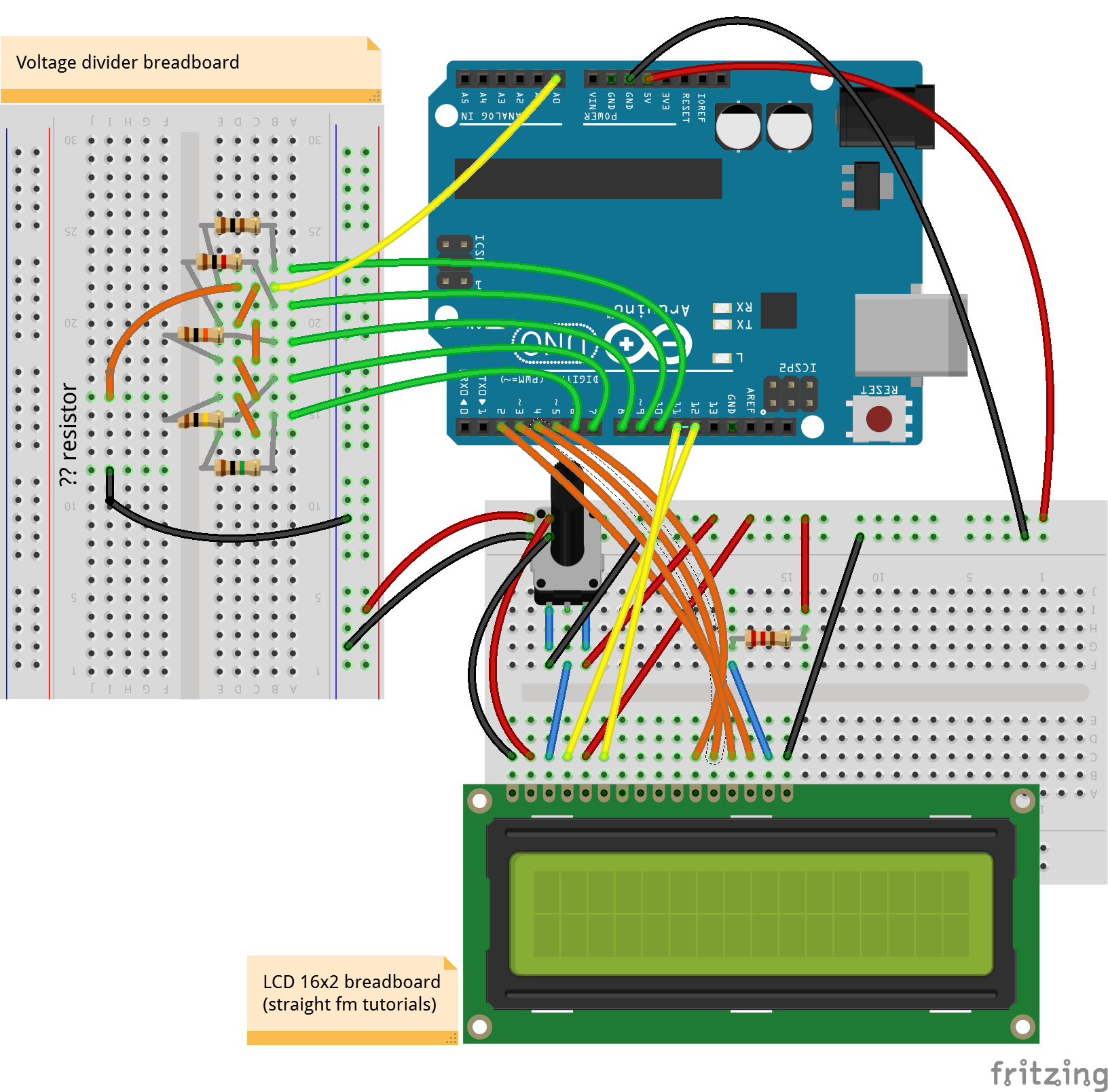## Comments

#### Published on

February 25, 2020

#### Members who respect this project

See similar projects
you might like

#### Sorting Resistors the Lazy Way

Project tutorial by Federico Vivaldi

• 18,649 views
• 40 comments
• 99 respects

#### V2 View direct Resistors values in accodance tthe color code

Project in progress by rafu

• 2,663 views
• 2 comments
• 8 respects

#### Add WiFi to Arduino UNO

• 466,354 views
• 127 comments
• 269 respects

#### Arduino Ohm Meter

Project showcase by lucian_vdo

• 11,828 views
• 5 comments
• 19 respects

#### Arduino Project: Burglar Zone Input Tester

Project tutorial by Cezarjar

• 3,569 views
• 2 comments
• 9 respects

#### Solar Charged Battery Powered Arduino Uno

Project in progress by Igor Fonseca Albuquerque

• 142,465 views
• 58 comments
• 213 respects